Processing ......FreeComputerBooks.com Links to Free Computer, Mathematics, Technical Books all over the World

Reinforcement Learning and Optimal Control
🌠 Top Free Machine Learning Books - 100% Free or Open Source!
• Title Reinforcement Learning and Optimal Control
• Author(s) Dimitri P. Bertsekas
• Publisher: Athena Scientific 2019
• Hardcover/Paperback: 276 pages
• eBook: PDF files
• Language: English
• ISBN-10: N/A
• ISBN-13: N/A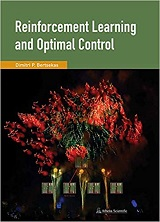Book Description

Reinforcement Learning (RL), one of the most active research areas in artificial intelligence, is a computational approach to learning whereby an agent tries to maximize the total amount of reward it receives while interacting with a complex, uncertain environment.

The purpose of the book is to consider large and challenging multistage decision problems, which can be solved in principle by dynamic programming and optimal control, but their exact solution is computationally intractable.

We discuss solution methods that rely on approximations to produce suboptimal policies with adequate performance. These methods are collectively referred to as reinforcement learning, and also by alternative names such as approximate dynamic programming, and neuro-dynamic programming.

The mathematical style of the book is somewhat different from the author's dynamic programming books, and the neuro-dynamic programming monograph, written jointly with John Tsitsiklis.

We rely more on intuitive explanations and less on proof-based insights. Still we provide a rigorous short account of the theory of finite and infinite horizon dynamic programming, and some basic approximation methods, in an appendix. For this we require a modest mathematical background: calculus, elementary probability, and a minimal use of matrix-vector algebra.

• Dimitri P. Bertsekas is an applied mathematician, electrical engineer, and computer scientist, and a professor at the department of Electrical Engineering and Computer Science in School of Engineering at the Massachusetts Institute of Technology (MIT), Cambridge, Massachusetts.
Reviews, Ratings, and Recommendations: Related Book Categories: Read and Download Links:Similar Books:
•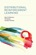Distributional Reinforcement Learning (Marc G. Bellemare, et al)

Distributional reinforcement learning is a new mathematical formalism for thinking about decisions. This first comprehensive guide provides a new mathematical formalism for thinking about decisions from a probabilistic perspective.

•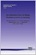An Introduction to Deep Reinforcement Learning

Deep reinforcement learning is the combination of reinforcement learning (RL) and deep learning. This field of research has recently been able to solve a wide range of complex decision-making tasks that were previously out of reach for a machine.

•Reinforcement Learning: An Introduction, Second Edition

It provides a clear and simple account of the key ideas and algorithms of reinforcement learning that is accessible to readers in all the related disciplines. Focuses on core online learning algorithms, with the more mathematical material set off in shaded boxes.

•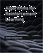Algorithms for Reinforcement Learning (Csaba Szepesvari)

This book focuses on those algorithms of reinforcement learning that build on the powerful theory of dynamic programming. It gives a fairly comprehensive catalog of learning problems, describe the core ideas, note a large number of state of the art algorithms, followed by the discussion of their theoretical properties and limitations.

•The Hundred-Page Machine Learning Book (Andriy Burkov)

Everything you really need to know in Machine Learning in a hundred pages! This book provides a great practical guide to get started and execute on ML within a few days without necessarily knowing much about ML apriori.

•Understanding Machine Learning: From Theory to Algorithms

Explains the principles behind the automated learning approach and the considerations underlying its usage. Provides an extensive theoretical account of the fundamental ideas underlying machine learning and the mathematical derivations.

•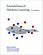Foundations of Machine Learning (Mehryar Mohri, et al)

This book is a general introduction to machine learning. It covers fundamental modern topics in machine learning while providing the theoretical basis and conceptual tools needed for the discussion and justification of algorithms.

•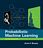Probabilistic Machine Learning: An Introduction (Kevin Murphy)

This book is a comprehensive introduction to machine learning that uses probabilistic models and inference as a unifying approach. It is written in an informal, accessible style, complete with pseudo-code for the most important algorithms.

•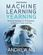Machine Learning Yearning (Andrew Ng)

You will learn how to align on ML strategies in a team setting, as well as how to set up development (dev) sets and test sets. After finishing this book, you will have a deep understanding of how to set technical direction for a machine learning project.

Book Categories
 :All CategoriesTop Free BooksRecent BooksMiscellaneous BooksComputer EngineeringComputer LanguagesComputer ScienceData Science/DatabasesJava and Java EE (J2EE)Linux and UnixMathematicsMicrosoft and .NETMobile ComputingNetworking and CommunicationsSoftware EngineeringSpecial TopicsWeb Programming
Other Categories